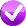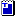# University of Washington, AP/CS A

## Lab 5: while loops

Except where otherwise noted, the contents of this document are Copyright 2013 Stuart Reges and Marty Stepp.

lab document created by Marty Stepp, Stuart Reges and Whitaker Brand

# Today's lab

Goals for this lab:

• use `while` loops for indefinite repetition
• Where you see this icon, you can click it to check the problem in Practice-It!# `while` Loops

`for` loops are fantastic for when we know how many times we want to repeat something. But sometimes we won't know how many times we'll want to repeat something in advance! `while` loops repeat indefinitely while a given condition is true.

```while (test) {
statement(s);
}
```

Example:

```int num = 1;
while (num < 5) {
System.out.print(num + " ");     // output: 1 2 3 4
num++;
}
```

# Exercise : `while` loop basics

Consider the following loop.

```int x = 1;
System.out.print(x);
while (x < 100) {
x = x + x;
System.out.print(", " + x);
}
```
 How many times does the code in the `while` loop execute? 7 What output is produced by the overall code? 1, 2, 4, 8, 16, 32, 64, 128

# Exercise : `while` loop mysteryFill in the boxes at right with the output produced by each method call.

```public static void mystery(int x) {
int y = 1;
int z = 0;
while (2 * y <= x) {
y = y * 2;
z++;
}
System.out.println(y + " " + z);
}
```
 `mystery(1);` `1 0` `mystery(6);` `4 2` `mystery(19);` `16 4` `mystery(39);` `32 5` `mystery(74);` `64 6`

# Exercise : `while` loop mysteryFill in the boxes at right with the output produced by each method call.

```public static void mystery2(int x, int y) {
int z = 0;
while (x % y != 0) {
x = x / y;
z++;
System.out.print(x + ", ");
}

System.out.println(z);
}
```
 `mystery2(25, 2);` `12, 1` `mystery2(32, 4);` `0` `mystery2(10345, 10);` `1034, 103, 10, 3` `mystery2(63, 2);` `31, 15, 7, 3, 1, 0, 6`

# Exercise : `while` loop mysteryFill in the boxes at right with the output produced by each method call.

```public static void mystery3(int x) {
int y = 0;
while (x % 2 == 0) {
y++;
x = x / 2;
}
System.out.println(x + " " + y);
}
```
 `mystery3(19);` `19 0` `mystery3(42);` `21 1` `mystery3(48);` `3 4` `mystery3(40);` `5 3` `mystery3(64);` `1 6`

# Exercise : `while` loop mystery

Fill in the boxes at right with the output produced by each method call. (Review how to do this type of problem in lab 5!)

```public static void mystery4(int n) {
int x = 1;
int y = 2;
while (y < n) {
if (n % y == 0) {
n = n / y;
x++;
} else {
y++;
}
}
System.out.println(x + " " + n);
}
```
 `mystery4(2);` `1 2` `mystery4(5);` `1 5` `mystery4(24);` `4 3` `mystery4(28);` `3 7`

# Exercise : assertionsWrite always/never/sometimes `true` at each point. If unsure how to, check out the assertions tutorial!

`x > y` `z == 0` `x == y`
A
B
C
D
E
```public static void mystery(int x, int y) {
int z = 0;

// Point A
while (x != y) {
// Point B
z++;
if (x > y) {
// Point C
x = x / 10;
} else {
// Point D
y = y / 10;
}
}

// Point E
System.out.println(x + " " + y + " " + z);
}```

You can also solve this problem in Practice-It by clicking on the check-mark above.

# Exercise : allDigitsOddWrite a method named `allDigitsOdd` that returns whether every digit of a positive integer is odd. Your method should return `true` if the number consists entirely of odd digits and `false` if any of its digits are even. 0, 2, 4, 6, and 8 are even digits, and 1, 3, 5, 7, 9 are odd digits.

For example, `allDigitsOdd(135319)` returns `true` but `allDigitsOdd(9145293)` returns `false`.

Hint: You can pull apart a number into its digits using ```/ 10``` and `% 10`.

# Exercise : firstDigit• Write a method named `firstDigit` that returns the first digit of an integer. For example, `firstDigit(3572)` should return `3`. It should work for negative numbers as well. For example, `firstDigit(-947)` should return `9`.
• Try solving this problem in Practice-It! using the link above.
• (Hint: This problem requires a loop.)

# Exercise : digitsInARowWrite a static method called `digitsInARow` that takes an integer `n` as a parameter and that returns the highest number of digits that appear in a row in the base-10 representation of `n`. For many numbers the answer will be 1 because they don't have adjacent digits that match. But for a number like 3555585, the answer is 4 because there are four occurrences of the digit 5 that appear in a row. Below are sample calls on the method.

```Method                  Value          Method                  Value
Call                  Returned         Call                  Returned
-------------------------------        -------------------------------
digitsInARow(0)           1            digitsInARow(8823)        2
digitsInARow(18)          1            digitsInARow(777)         3
digitsInARow(394)         1            digitsInARow(82888)       3
digitsInARow(99)          2            digitsInARow(7111171)     4
digitsInARow(8229)        2            digitsInARow(233333888)   5
```

You are not allowed to use a string to solve this problem. You may assume that the value passed to the method is greater than or equal to 0.

Hint: You can pull apart a number into its digits using ```/ 10``` and `% 10`.

Solve this problem in Practice-It by clicking on the check-mark above.

# Exercise : digitSumWrite a method named `digitSum` that accepts an integer as a parameter and returns the sum of the digits of that number. For example, the call `digitSum(29107)` returns 2+9+1+0+7 or 19. For negative numbers, return the same value that would result if the number were positive. For example, `digitSum(-456)` returns 4+5+6 or 15. The call `digitSum(0)` returns 0.

(Hint: This is a cumulative algorithm. To extract a digit from a number, use `/ 10` and `% 10` operations.)

# Exercise : flipWrite a method `flip` that takes a `Random` object as a parameter and that prints information about a coin-flipping simulation.

Your method should use the `Random` object to produce a sequence of simulated coin flips, printing whether each flip comes up "heads" or "tails". Each outcome should be equally likely. Your method should stop flipping when you see three heads in a row.

Solve this problem in Practice-It by clicking on the check-mark above.

# Exercise : makeGuessesWrite a method named `makeGuesses` that will output random numbers between 1 and 50 inclusive until it outputs one of at least 48. Output each guess and the total number of guesses made. Below is a sample execution:

```guess = 43
guess = 47
guess = 45
guess = 27
guess = 49
total guesses = 5
```

If you're not sure how make/use a Random, review them here.

# Exercise : printAverage• Write a method named `printAverage` that accepts a `Scanner` for the console as a parameter and repeatedly prompts the user for numbers. Once any number less than zero is typed, the average of all non-negative numbers typed is displayed. Display the average as a double, and do not round it. For example, a call to your method might look like this:
```Scanner console = new Scanner(System.in);
printAverage(console);
```
The following is one example log of execution for your method:
```Type a number: 10
Type a number: 15
Type a number: -1
Average was 12.5
```
If the first number typed is negative, do not print an average.
• Try solving this problem in Practice-It! using the link above.
• (Hint: To find the average, you will need to know both the sum and a count of the number of numbers to average.)

# Exercise : ProcessName

Copy/paste the following code into jGRASP, ordownload it. Then go to the next slide.

```import java.util.*;  // for Scanner

public class ProcessName {
public static void main(String[] args) {
Scanner console = new Scanner(System.in);

// read the entire input as a single line
String input = console.nextLine();

System.out.println("Your name is: " + name);
}
}
```

continued on the next slide ...

# Exercise - code to add

Add code to the program so that it reads the user's first and last name (reading the entire line of input as a single string), then prints the last name followed by a comma and the first initial. Assume that the user types a single first name, a space, and then a single last name.

Example:
```Type your name: Jessica Miller
```
If you get stuck, ask a TA for help!

# Exercise : ProcessName Solution

```import java.util.*;  // for Scanner

public class ProcessName {
public static void main(String[] args) {
Scanner console = new Scanner(System.in);

// read the entire input as a single line
String input = console.nextLine();

int spaceIndex = input.indexOf(" ");
String lastName = input.substring(spaceIndex + 1);
char firstInitial = input.charAt(0);

String name = lastName + ", " + firstInitial + ".";

System.out.println("Your name is: " + name);
}
}```

# Exercise : ProcessName2Modify your previous `ProcessName` program so that it re-prompts until the user types a name that is at least 5 letters total in length and has at least one space in it. Example:

```Type your name: Joe
Error, must be at least 5 chars with a space.